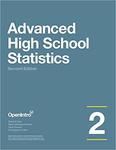## Open Textbooks#### Title

Advanced High School Statistics - 2nd Edition

#### Contributors

David Diez - Author / Christopher Barr - Author / Mine Çetinkaya-Rundel - Author / Leah Dorazio - Author

#### Description

We hope readers will take away three ideas from this book in addition to forming a foundationof statistical thinking and methods. (1) Statistics is an applied field with a wide range of practical applications. (2) You don't have to be a math guru to learn from real/ interesting data. (3) Data are messy/ and statistical tools are imperfect. But/ when you understand the strengths and weaknesses of these tools/ you can use them to learn about the real world. Textbook overviewThe chapters of this book are as follows: 1. Data collection. Data structures/ variables/ and basic data collection techniques. 2. Summarizing data. Data summaries and graphics. 3. Probability. The basic principles of probability. 4. Distributions of random variables. Introduction to key distributions/ and how the normal model applies to the sample mean and sample proportion. 5. Foundation for inference. General ideas for statistical inference in the context of estimating the population proportion. 6. Inference for categorical data. Inference for proportions using the normal and chisquare distributions. 7. Inference for numerical data. Inference for one or two sample means using the t distribution/ and comparisons of many means using ANOVA. 8. Introduction to linear regression. An introduction to regression with two variables. Instructions are also provided in several sections for using Casio and TI calculators.

#### Subject 1

Mathematics - Applied

#### Subject 2

Mathematics - Applied

OpenIntro

#### Resources

Open Textbook Library